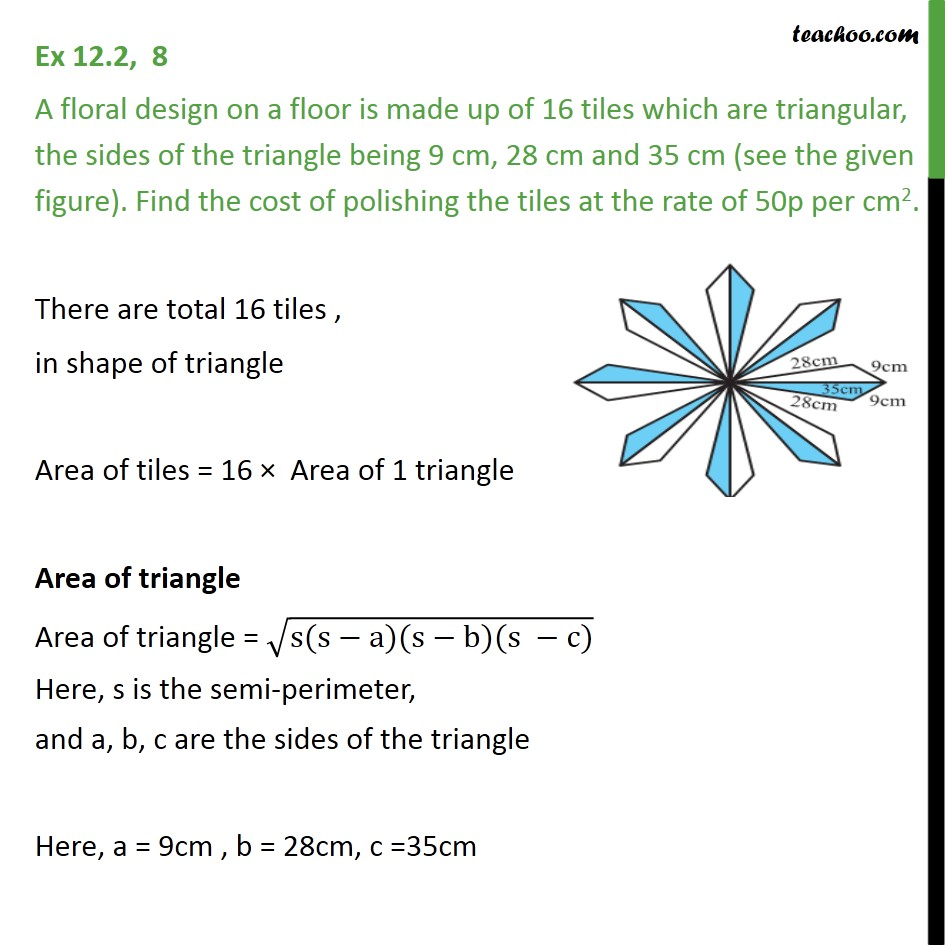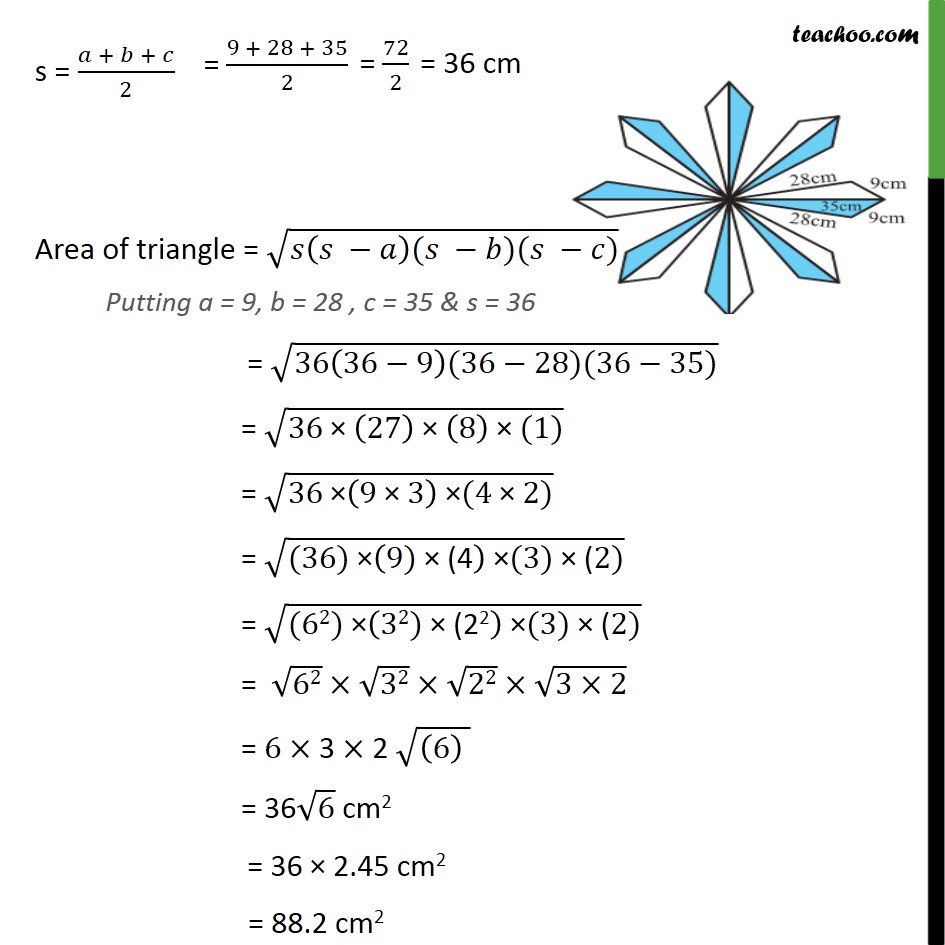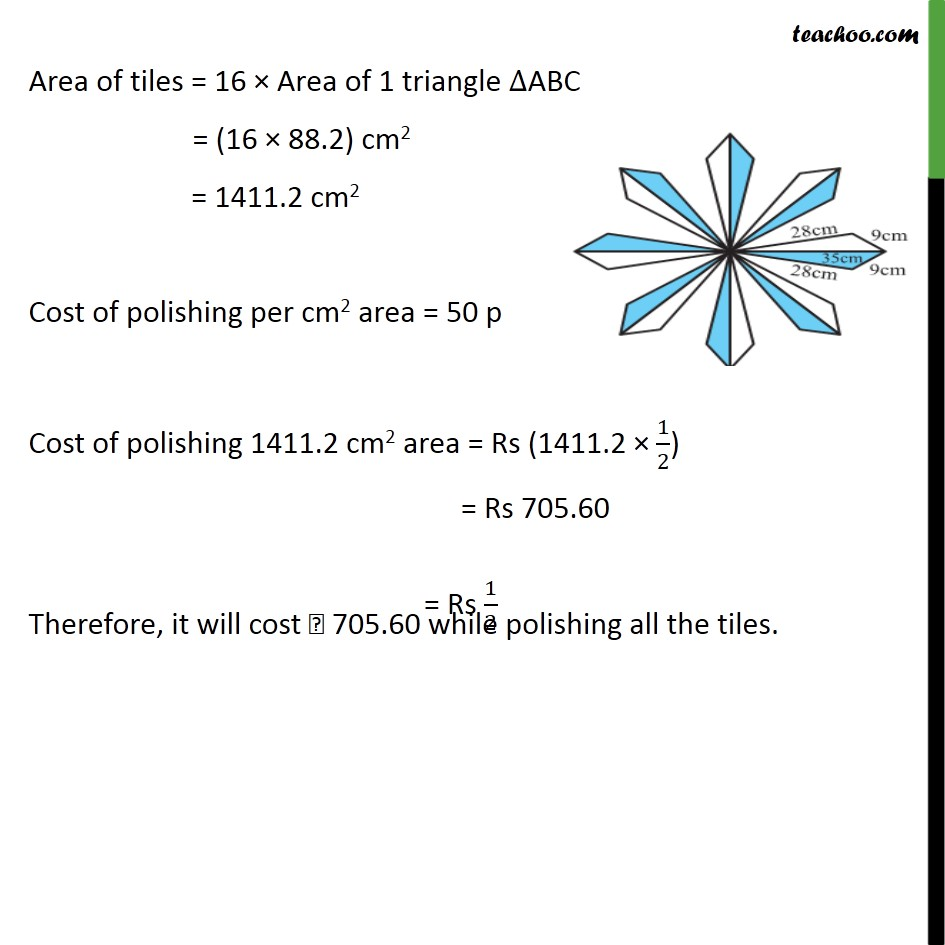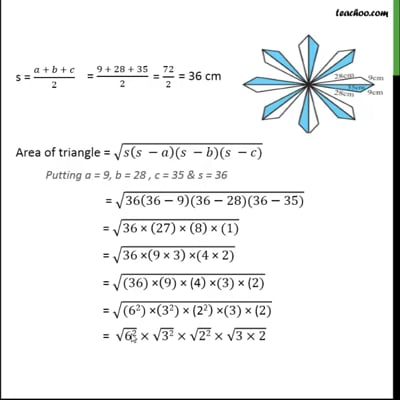Ex 12.2

Chapter 12 Class 9 Herons Formula (Term 1)
Serial order wiseThis video is only available for Teachoo black users

### Transcript

Ex 12.2, 8 A floral design on a floor is made up of 16 tiles which are triangular, the sides of the triangle being 9 cm, 28 cm and 35 cm (see the given figure). Find the cost of polishing the tiles at the rate of 50p per cm2. There are total 16 tiles , in shape of triangle Area of tiles = 16 Area of 1 triangle Area of triangle Area of triangle = (s(s a)(s b)(s c)) Here, s is the semi-perimeter, and a, b, c are the sides of the triangle Here, a = 9cm , b = 28cm, c =35cm s = ( + + )/2 Area of triangle = ( ( )( )( )) Putting a = 9, b = 28 , c = 35 & s = 36 = (36(36 9)(36 28)(36 35)) = (36" " (27) " " (8)" " (1)) = (36" " (9" " 3) " " (4" " 2)) = ((36)" " (9)" (4" ) " " (3)" (" 2)) = ((62)" " (32)" (22" ) " " (3)" (" 2)) = 62 32 22 (3 2) = 6 3 2 ((6) ) = 36 6 cm2 = 36 2.45 cm2 = 88.2 cm2 Area of tiles = 16 Area of 1 triangle ABC = (16 88.2) cm2 = 1411.2 cm2 Cost of polishing per cm2 area = 50 p Cost of polishing 1411.2 cm2 area = Rs (1411.2 1/2) = Rs 705.60 Therefore, it will cost 705.60 while polishing all the tiles.